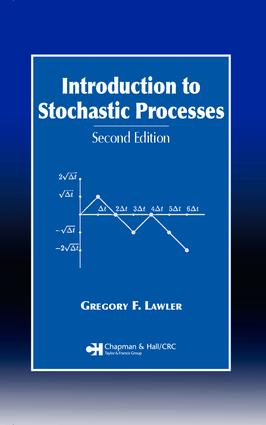Introduction to Stochastic Processes

2nd Edition

Chapman and Hall/CRC

248 pages | 13 B/W Illus.

Purchasing Options:\$ = USD
Hardback: 9781584886518
pub: 2006-05-16
SAVE ~\$22.00
\$110.00
\$88.00
x
eBook (VitalSource) : 9781315273600
pub: 2018-10-03
from \$55.00

FREE Standard Shipping!

Description

Emphasizing fundamental mathematical ideas rather than proofs, Introduction to Stochastic Processes, Second Edition provides quick access to important foundations of probability theory applicable to problems in many fields. Assuming that you have a reasonable level of computer literacy, the ability to write simple programs, and the access to software for linear algebra computations, the author approaches the problems and theorems with a focus on stochastic processes evolving with time, rather than a particular emphasis on measure theory.

For those lacking in exposure to linear differential and difference equations, the author begins with a brief introduction to these concepts. He proceeds to discuss Markov chains, optimal stopping, martingales, and Brownian motion. The book concludes with a chapter on stochastic integration. The author supplies many basic, general examples and provides exercises at the end of each chapter.

New to the Second Edition:

• Expanded chapter on stochastic integration that introduces modern mathematical finance

• Introduction of Girsanov transformation and the Feynman-Kac formula

• Expanded discussion of Itô's formula and the Black-Scholes formula for pricing options

• New topics such as Doob's maximal inequality and a discussion on self similarity in the chapter on Brownian motion

Applicable to the fields of mathematics, statistics, and engineering as well as computer science, economics, business, biological science, psychology, and engineering, this concise introduction is an excellent resource both for students and professionals.

Preface to Second Edition

Preface to First Edition

PRELIMINARIES

Introduction

Linear Differential Equations

Linear Difference Equations

Exercises

FINITE MARKOV CHAINS

Definitions and Examples

Large-Time Behavior and Invariant Probability

Classification of States

Return Times

Transient States

Examples

Exercises

COUNTABLE MARKOV CHAINS

Introduction

Recurrence and Transience

Positive Recurrence and Null Recurrence

Branching Process

Exercises

CONTINUOUS-TIME MARKOV CHAINS

Poisson Process

Finite State Space

Birth-and-Death Processes

General Case

Exercises

OPTIMAL STOPPING

Optimal Stopping of Markov Chains

Optimal Stopping with Cost

Optimal Stopping with Discounting

Exercises

MARTINGALES

Conditional Expectation

Definition and Examples

Optional Sampling Theorem

Uniform Integrability

Martingale Convergence Theorem

Maximal Inequalities

Exercises

RENEWAL PROCESSES

Introduction

Renewal Equation

Discrete Renewal Processes

M/G/1 and G/M/1 Queues

Exercises

REVERSIBLE MARKOV CHAINS

Reversible Processes

Convergence to Equilibrium

Markov Chain Algorithms

A Criterion for Recurrence

Exercises

BROWNIAN MOTION

Introduction

Markov Property

Zero Set of Brownian Motion

Brownian Motion in Several Dimensions

Recurrence and Transience

Fractal Nature of Brownian Motion

Scaling Rules

Brownian Motion with Drift

Exercises

STOCHASTIC INTEGRATION

Integration with Respect to Random Walk

Integration with Respect to Brownian Motion

Itô's Formula

Extensions if Itô's Formula

Continuous Martingales

Girsanov Transformation

Feynman-Kac Formula

Black-Scholes Formula

Simulation

Exercises

Suggestions for Further Reading

Index

Greogory F. Lawler